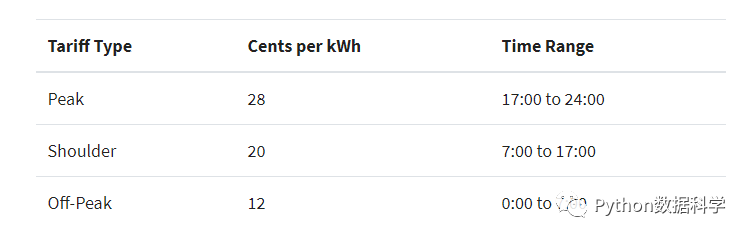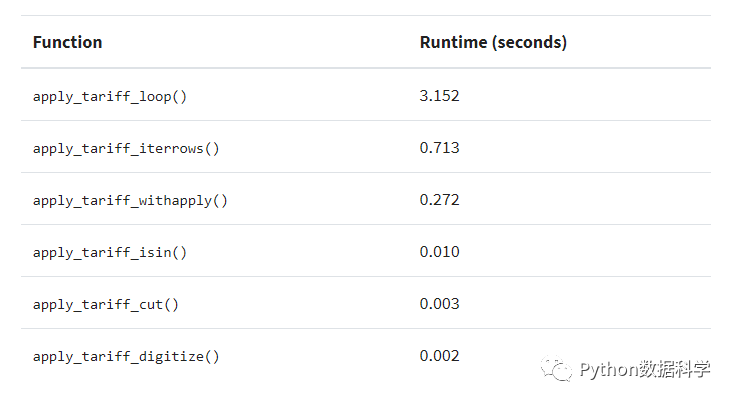用这几个方法提高pandas运行速度“快速，灵活，富有表现力的数据结构，旨在使”关系“或”标记“数据的使用既简单又直观。”

pandas是基于numpy库的数组结构上构建的，并且它的很多操作都是（通过numpy或者pandas自身由Cpython实现并编译成C的扩展模块）在C语言中实现的。因此，如果正确使用pandas的话，它的运行速度应该是非常快的。

• 将datetime数据与时间序列一起使用的优点

• 进行批量计算的最有效途径

• 通过HDFStore存储数据节省时间

>>> import pandas as pd
>>pd.__version__
'0.23.1'

# 导入数据集
date_time  energy_kwh
0  1/1/13 0:00       0.586
1  1/1/13 1:00       0.580
2  1/1/13 2:00       0.572
3  1/1/13 3:00       0.596
4  1/1/13 4:00       0.592

>>> df.dtypes
date_time      object
energy_kwh    float64
dtype: object

>>type(df.iat)
str

object 类型像一个大的容器，不仅仅可以承载 str，也可以包含那些不能很好地融进一个数据类型的任何特征列。而如果我们将日期作为 str 类型就会极大的影响效率。

>>> df['date_time'] = pd.to_datetime(df['date_time'])
>>> df['date_time'].dtype
datetime64[ns]

date_time    energy_kwh
0    2013-01-01 00:00:00         0.586
1    2013-01-01 01:00:00         0.580
2    2013-01-01 02:00:00         0.572
3    2013-01-01 03:00:00         0.596
4    2013-01-01 04:00:00         0.592

date_time的格式已经自动转化了，但这还没完，在这个基础上，我们还是可以继续提高运行速度的。如何提速呢？为了更好的对比，我们首先通过 timeit 装饰器来测试一下上面代码的转化时间。

>>> @timeit(repeat=3, number=10)
... def convert(df, column_name):
...     return pd.to_datetime(df[column_name])

>>> df['date_time'] = convert(df, 'date_time')
Best of 3 trials with 10 function calls per trial:
Function convert ran in average of 1.610 seconds.

1.61s，看上去挺快，但其实可以更快，我们来看一下下面的方法。

>>> @timeit(repeat=3, number=100)
>>def convert_with_format(df, column_name):
...     return pd.to_datetime(df[column_name],
...                           format='%d/%m/%y %H:%M')
Best of 3 trials with 100 function calls per trial:
Function convert_with_format ran in average of 0.032 seconds.

pandas数据的循环操作def apply_tariff(kwh, hour):

"""计算每个小时的电费"""
if 0 <= hour < 7:
rate = 12
elif 7 <= hour < 17:
rate = 20
elif 17 <= hour < 24:
rate = 28
else:
raise ValueError(f'Invalid hour: {hour}')
return rate * kwh

>>> # 不赞同这种操作
>>> @timeit(repeat=3, number=100)
... def apply_tariff_loop(df):
...     """Calculate costs in loop.  Modifies df inplace."""
...     energy_cost_list = []
...     for i in range(len(df)):
...
# 获取用电量和时间（小时）
...         energy_used = df.iloc[i]['energy_kwh']
...         hour = df.iloc[i]['date_time'].hour
...         energy_cost = apply_tariff(energy_used, hour)
...         energy_cost_list.append(energy_cost)
...     df['cost_cents'] = energy_cost_list
...
>>> apply_tariff_loop(df)
Best of 3 trials with 100 function calls per trial:
Function apply_tariff_loop ran in average of
3.152 seconds.

• 首先，它需要初始化一个将记录输出的列表。

• 其次，它使用不透明对象范围(0，len(df))循环，然后在应用apply_tariff()之后，它必须将结果附加到用于创建新DataFrame列的列表中。它还使用df.iloc [i] ['date_time']执行所谓的链式索引，这通常会导致意外的结果。

• 但这种方法的最大问题是计算的时间成本。对于8760行数据，此循环花费了3秒钟。接下来，你将看到一些改进的Pandas结构迭代解决方案。

.itertuples为每一行产生一个namedtuple，并且行的索引值作为元组的第一个元素。nametuple是Python的collections模块中的一种数据结构，其行为类似于Python元组，但具有可通过属性查找访问的字段。

.iterrows为DataFrame中的每一行产生（index，series）这样的元组。

>>> @timeit(repeat=3, number=100)
... def apply_tariff_iterrows(df):
...     energy_cost_list = []
...     for index, row in df.iterrows():
...
获取用电量和时间（小时）
...         energy_used = row['energy_kwh']
...         hour = row['date_time'].hour
...         # 添加cost列表
...         energy_cost = apply_tariff(energy_used, hour)
...         energy_cost_list.append(energy_cost)
...     df['cost_cents'] = energy_cost_list
...
>>> apply_tariff_iterrows(df)
Best of 3 trials with 100 function calls per trial:
Function apply_tariff_iterrows ran in average of
0.713 seconds.

Pandas的 .apply()方法

>>> @timeit(repeat=3, number=100)
... def apply_tariff_withapply(df):
...     df['cost_cents'] = df.apply(
...         lambda row: apply_tariff(
...             kwh=row['energy_kwh'],
...             hour=row['date_time'].hour),
...         axis=1)
...
>>> apply_tariff_withapply(df)
Best of 3 trials with 100 function calls per trial:
Function apply_tariff_withapply ran in average of
0.272 seconds.

.apply的语法优点很明显，行数少，代码可读性高。在这种情况下，所花费的时间大约是.iterrows方法的一半。

df.set_index('date_time', inplace=True)

@timeit(repeat=3, number=100)
def apply_tariff_isin(df):

# 定义小时范围Boolean数组
peak_hours = df.index.hour.isin(range(1724))
shoulder_hours = df.index.hour.isin(range(717))
off_peak_hours = df.index.hour.isin(range(07))

# 使用上面的定义
df.loc[peak_hours, 'cost_cents'] = df.loc[peak_hours, 'energy_kwh'] * 28
df.loc[shoulder_hours,'cost_cents'] = df.loc[shoulder_hours, 'energy_kwh'] * 20
df.loc[off_peak_hours,'cost_cents'] = df.loc[off_peak_hours, 'energy_kwh'] * 12

>>> apply_tariff_isin(df)
Best of 3 trials with 100 function calls per trial:
Function apply_tariff_isin ran in average
of 0.010 seconds.

[False, False, False, ..., True, True, True]

@timeit(repeat=3, number=100)
def apply_tariff_cut(df):
cents_per_kwh = pd.cut(x=df.index.hour,
bins=,
include_lowest=True,
labels=).astype(int)
df['cost_cents'] = cents_per_kwh * df['energy_kwh']

>>> apply_tariff_cut(df)
Best of 3 trials with 100 function calls per trial:
Function apply_tariff_cut ran in average
of 0.003 seconds.

@timeit(repeat=3, number=100)
def apply_tariff_digitize(df):
prices = np.array()
bins = np.digitize(df.index.hour.values, bins=)
df['cost_cents'] = prices[bins] * df['energy_kwh'].values

>>> apply_tariff_digitize(df)
Best of 3 trials with 100 function calls per trial:
Function apply_tariff_digitize ran in average
of 0.002 seconds.

1. 使用向量化操作：没有for循环的Pandas方法和函数。

2. 将.apply方法：与可调用方法一起使用。

3. 使用.itertuples：从Python的集合模块迭代DataFrame行作为namedTuples。

4. 使用.iterrows：迭代DataFrame行作为(index，Series)对。虽然Pandas系列是一种灵活的数据结构，但将每一行构建到一个系列中然后访问它可能会很昂贵。

5. 使用“element-by-element”循环：使用df.loc或df.iloc一次更新一个单元格或行。Pandas有一个内置的解决方案，它使用 HDF5，这是一种专门用于存储表格数据阵列的高性能存储格式。 Pandas的 HDFStore 类允许你将DataFrame存储在HDF5文件中，以便可以有效地访问它，同时仍保留列类型和其他元数据。它是一个类似字典的类，因此您可以像读取Python dict对象一样进行读写。

# 创建储存对象，并存为 processed_data
data_store = pd.HDFStore('processed_data.h5')

# 将 DataFrame 放进对象中，并设置 key 为 preprocessed_df
data_store['preprocessed_df'] = df
data_store.close()

# 获取数据储存对象
data_store = pd.HDFStore('processed_data.h5')

# 通过key获取数据
preprocessed_df = data_store['preprocessed_df']
data_store.close()

• 尝试尽可能使用矢量化操作，而不是在df 中解决for x的问题。如果你的代码是许多for循环，那么它可能更适合使用本机Python数据结构，因为Pandas会带来很多开销。

• 如果你有更复杂的操作，其中矢量化根本不可能或太难以有效地解决，请使用.apply方法。

• 如果必须循环遍历数组（确实发生了这种情况），请使用.iterrows()或.itertuples()来提高速度和语法。

• Pandas有很多可选性，几乎总有几种方法可以从A到B。请注意这一点，比较不同方法的执行方式，并选择在项目环境中效果最佳的路线。

• 一旦建立了数据清理脚本，就可以通过使用HDFStore存储中间结果来避免重新处理。

• 将NumPy集成到Pandas操作中通常可以提高速度并简化语法。▼ 点击成为社区注册会员          「在看」一下，一起PY！

12-172万+

05-011875
03-01131
12-05739
04-272218
01-05595
11-27100
11-1954
01-06304
11-2782
12-08386
01-29208
10-265198点击重新获取扫码支付余额充值